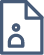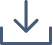### Proof Of Calculi Equivalence In The Hol Theorem Prover

The aim of this project is to prove the equivalence of sequent calculus, natural deduction and Hilbert calculus for classical propositional logic in the HOL theorem prover. HOL is an interactive theorem prover implemented in Standard ML, which uses Higher Order Logic as an environment to formalise proofs. The task will be broken down into smaller lemmas which will combine into a proof of equivalence. I will first prove equivalence in intuitionistic logic, and then move to classical. The theorem(s) can be stated: “There exists a proof of ? in calculus X if and only if there exists a proof of ? in calculus Y”, where ? is a provable formula in intuitionistic/classical logic. If time allows, I would like to also do the proofs for the first-order logic or some modal logic(s).#### Alexander Cox

Australian National University

Alexander (Alex) is a theoretical computer science student at the Australian National University. He is passionate about mathematical logic and mathematical models of computation (such as lambda calculus and automata). He also studies philosophy, focusing on moral, ethical and political philosophy and philosophy of science. In his spare time he enjoys spending time with his wife and going for walks.